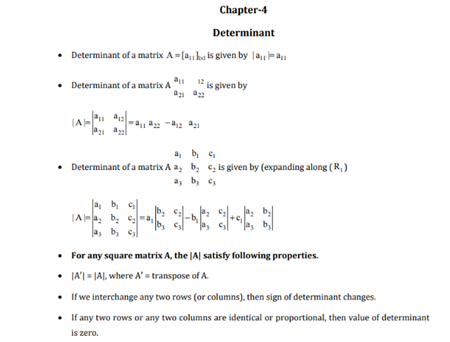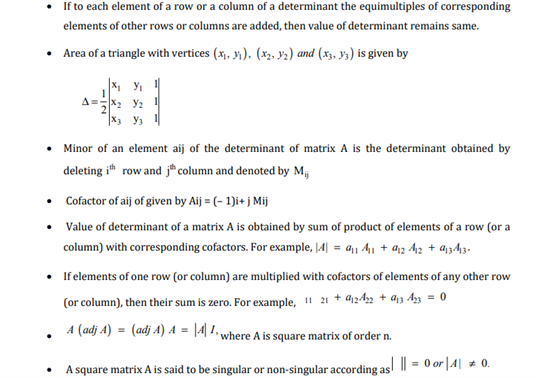# Maths Revision Notes for Class 12 Chapter 4 Determinants.

Determinants is the 4th chapter present in the class 12 maths syllabus, whose revision notes can be found here. We shall take a closer look into this chapter to gain a better understanding of the different topics and concepts that are introduced in Determinants. Some of the different topics that are useful for students in problem-solving are explained in this chapter, such as the linear equations format and the matrix format. On the other hand, the applications of determinants and its uses in different fields are also taught here. Learning the different types of determinants and properties is also important in preparing for the main Math exams.

Get the Revision Notes of chapter 4 Determinants below: